Triangle A triangle is a three-sided figure. The sum of the three angles in a triangle is always equal to 180°. Triangles are often classified by their sides. An equilateral triangle has 3 sides of equal length. An isosceles triangle has 2 sides of equal length. A scalene triangle has three sides of differing length. Triangles can also be classified by their angles: An acute triangle has all three angles less than 90°. A right triangle has one right angle (a 90° angle). An obtuse triangle has one angle greater than 90°. Each of these types of triangles is shown in Figure 1-15.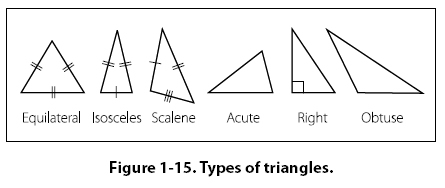The formula for the area of a triangle is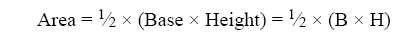Example: Find the area of the obtuse triangle shown in Figure 1-16. First, substitute the known values in the area formula. A = 1⁄2 x (B x H) = 1⁄2 x (2'6" x 3'2")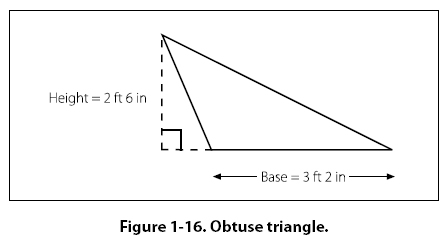Next, convert all dimensions to inches: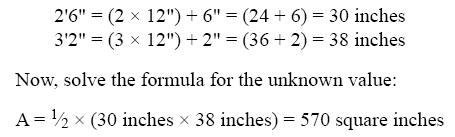Parallelogram A parallelogram is a four-sided figure with two pairs of parallel sides. [Figure 1-17] Parallelograms do not necessarily have four right angles. The formula for the area of a parallelogram is: Area = Length x Height = L x H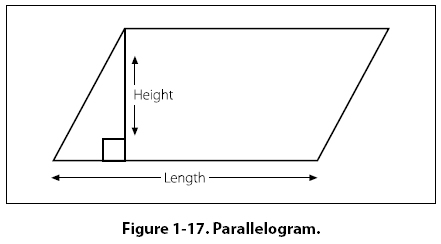Trapezoid A trapezoid is a four-sided figure with one pair of parallel sides. [Figure 1-18] The formula for the area of a trapezoid is: Area = 1/2 (Base1 + Base2) x Height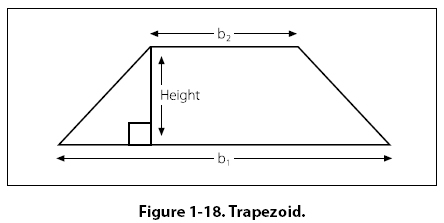Example: What is the area of a trapezoid in Figure 1-19 whose bases are 14 inches and 10 inches, and whose height (or altitude) is 6 inches? First, substitute the known values in the formula.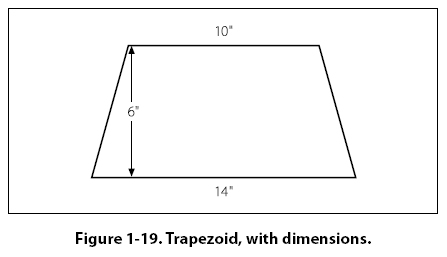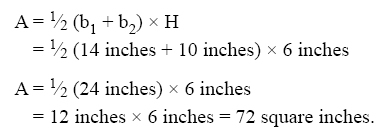©AvStop Online Magazine                                                                                                                                                      Contact Us              Return To Books